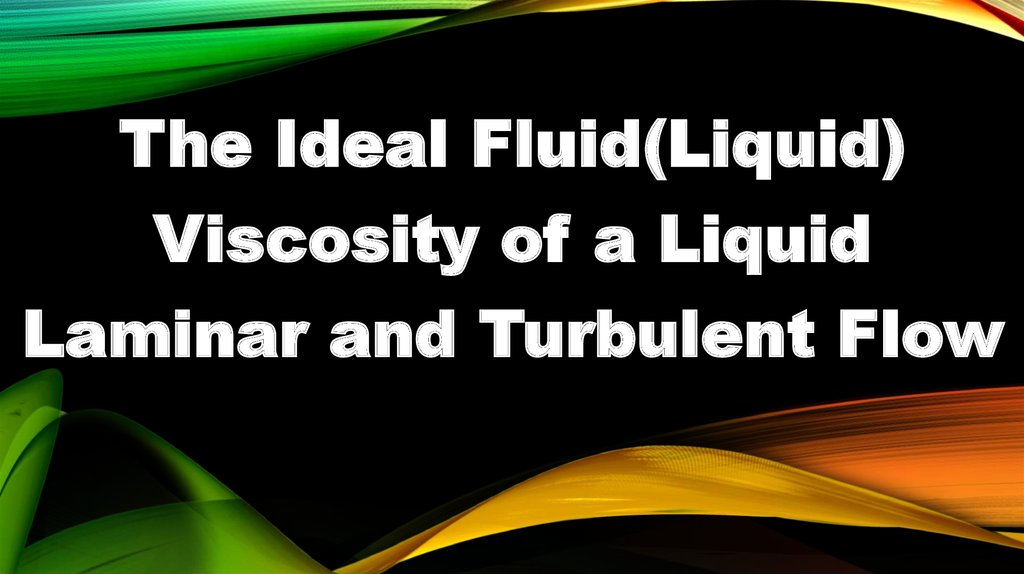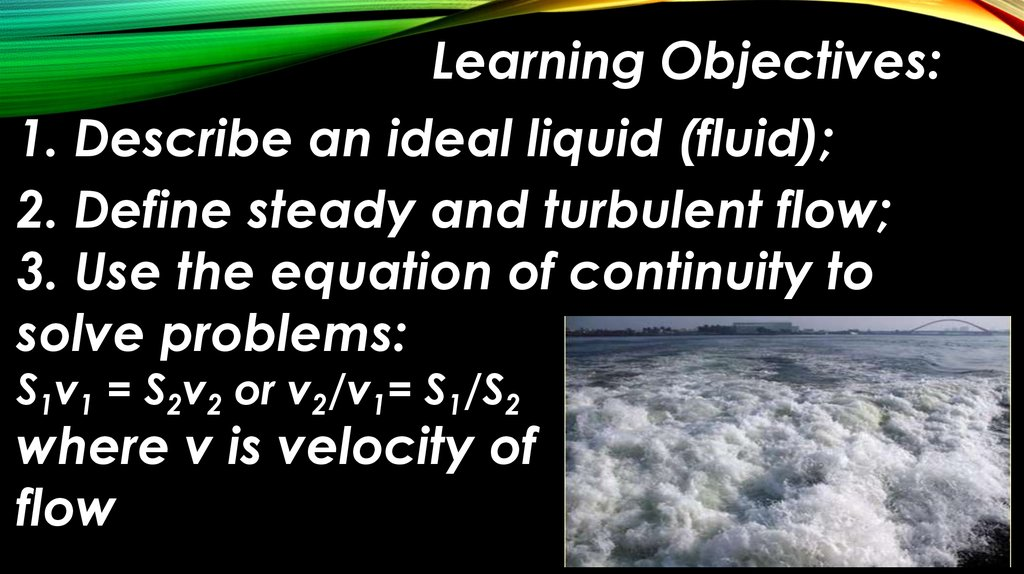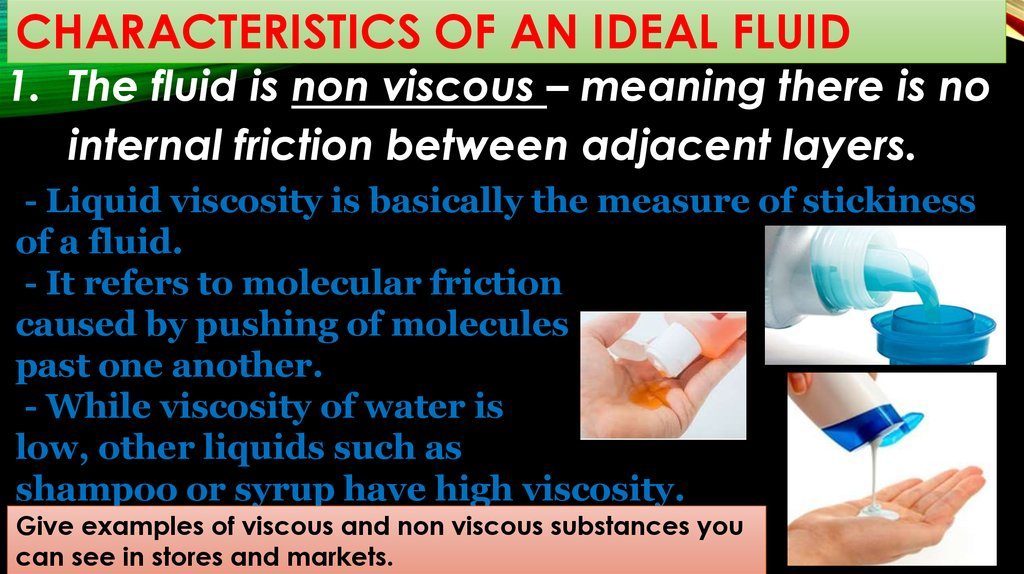The Ideal Fluid (Liquid) Viscosity of a Liquid Laminar and Turbulent Flow

1.

The Ideal Fluid(Liquid)
Viscosity of a Liquid
Laminar and Turbulent Flow

2.

Learning Objectives:
1. Describe an ideal liquid (fluid);
2. Define steady and turbulent flow;
3. Use the equation of continuity to
solve problems:
S1v1 = S2v2 or v2/v1= S1/S2
where v is velocity of
flow

3.

CHARACTERISTICS OF AN IDEAL FLUID
1. The fluid is non viscous – meaning there is no
- Liquid viscosity is basically the measure of stickiness
of a fluid.
- It refers to molecular friction
caused by pushing of molecules
past one another.
- While viscosity of water is
low, other liquids such as
shampoo or syrup have high viscosity.
Give examples of viscous and non viscous substances you
can see in stores and markets.

4.

CHARACTERISTICS OF AN IDEAL FLUID
Lava is an example
of a viscous
fluid.
The viscosity
decreases with
increasing
temperature: The
hotter the lava, the
more easily it can
flow.

5.

CHARACTERISTICS OF AN IDEAL FLUID
One factor that affects viscosity is flow conditions . The
two main flow conditions of a liquid are laminar and
turbulent.
- Laminar flow is characterized by the smooth
flow of the fluid in layers that do not mix.
- Turbulent flow, or turbulence, is
characterized by eddies and swirls
that mix layers of fluid together.

6.

The flow of smoke rising
from these incense sticks is laminar up to a certain
point, and then becomes turbulent.

7.

Smoke rises smoothly for a
while and then begins to form
swirls and eddies. The smooth
flow is called laminar flow,
whereas the swirls and eddies
typify turbulent flow. If you
watch the smoke (being careful
not to breathe on it), you will
notice that it rises more rapidly when flowing
smoothly than after it becomes turbulent, implying
that turbulence poses more resistance to flow.

8.

CHARACTERISTICS OF AN IDEAL FLUID
2. The fluid is incompressible – meaning its
density is constant as it flows or its volume
flows with the fluid velocity.

9.

CHARACTERISTICS OF AN IDEAL FLUID
3. The fluid is steady - a flow in which the
velocity, density and pressure of the fluid
at a particular fixed point does not change
with time.

10.

CHARACTERISTICS OF AN IDEAL FLUID
4. The fluid moves without turbulence –
meaning the flow is laminar.

11.

*VISCOUS FLOW
-In an ideal uid there is no
viscosity to hinder the uid
layers as they slide past one
another.
-Within a pipe of uniform
cross section, every layer
of an ideal uid moves with the same velocity,
even the layer next to the wall.

12.

*VISCOUS FLOW
- When viscosity is present, the uid layers have
different velocities.
- The uid at the center of
the pipe has the greatest
velocity.
- In contrast, the uid
layer next to the wall
surface does not move at
all, because it is held tightly by intermolecular
forces.

13.

To help introduce
viscosity in a
quantitative fashion, this
figure shows a viscous uid
between two parallel plates.
The top plate is free to
move, while the bottom one
is stationary.

14.

If the top plate is to move
with a velocity relative to
the bottom plate, a force
is required. For a highly
viscous uid, like thick
honey, a large force is
needed; for a less viscous
uid, like water, a smaller
one will do.

15.

This drawing
suggests, we
may imagine
the uid to be
composed of
many thin horizontal layers. When the top plate
moves, the intermediate uid layers slide over
each other. The velocity of each layer is
different, changing uniformly from at the top
plate to zero at the bottom plate.

16.

The resulting
ow is
called
laminar
ow, since a
thin layer is
often
referred to as a lamina. As each layer moves, it is
subjected to viscous forces from its neighbors.

17.

The amount of force
required to move the
top plate to a certain
velocity depends on
several factors.
Larger areas A, being
in contact with more
uid, require larger
forces, so that the force is proportional to the
contact area (F
A) .
How is the force needed to move the plate to a certain
velocity related to the area of the plate?

18.

For a given area,
greater speeds
require larger
forces, with the
result that the
force is
proportional to the
speed (F
v) .
How is the force required to move the plate to a certain
velocity related to velocity of the fluid?

19.

The force is also
inversely
proportional to the
perpendicular
distance y between
the top and bottom
plates (F
1/y) .
The larger the distance y, the smaller is the force
required to achieve a given speed with a given
contact area.

20.

These three proportionalities
can be expressed simultaneously in the following
way: F
Av/y.

22.

Notes:
- Values of viscosity depend on
the nature of the uid.
- Under ordinary conditions, the viscosities of
liquids are signi cantly larger than those of
gases.
- Viscosities of either liquids or gases depend
markedly on temperature.

23.

Notes:
- Usually, the viscosities of liquids decrease as
the temperature is increased.
- Anyone who has heated
honey or oil, for example,
knows that these uids ow
much more freely at an elevated temperature.
- In contrast, the viscosities of gases increase as
the temperature is raised.

24.

Notes:
Viscous ow occurs in a wide variety of
situations, such as
- oil moving through a pipeline
or a liquid being forced through
-the needle of a hypodermic
syringe.

25.

For viscous ow, the
difference in pressures
P2 - P1, the radius R and
length L of the tube, and
the viscosity η of the
uid in uence the
volume ow rate.

26.

Factors that determine the volume ow rate Q (in
m3/s) of the viscous uid:
1. Difference in pressures P2 - P1 must be
maintained between any two locations along the
pipe for the uid to ow. Q is proportional to P2 P1, a greater pressure diffe
ow rate.
2. A long pipe offers greater
resistance to the ow than a short pipe does, and
Q is inversely proportional to the length L.

27.

Factors that determine the volume ow rate Q (in
m3/s) of the viscous uid:
3. High-viscosity uids ow less readily than lowviscosity uids, and Q is inversely proportional to
the viscosity η.
4. The volume ow rate is larger in a pipe of
larger radius, Q being proportional to the fourth
4
power of the radius, or R . For instance, the pipe
radius is reduced to one-half of its original value,
the volume ow rate is reduced to one-sixteenth
of its original value

28.

The mathematical relation for Q in terms of these
parameters was discovered by Poiseuille and is known as
Poiseuille’s law.

29.

Example: Giving an Injection
A hypodermic syringe is lled with a solution whose
viscosity is 1.5 x 10 -3 Pa-s. As the figure shows, the
plunger area of the syringe is 8.0 x 10-5 m 2 , and the
length of the needle is 0.025 m. The internal radius of
the needle is 4.0 x 10-4 m.
The gauge pressure in a
vein is 1900 Pa (14 mm
of mercury). What force
must be applied to the
plunger, so that 1.0 x 10 -6 m 3
of solution can be injected in 3.0 s?